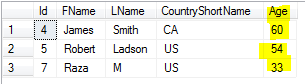## Scenario:

Let's say that we have dbo.Cutomer table and one of the column is Age. If we would like to return all the records from dbo.Customer table where age between 33 and 60. What Logical Operator we can use?

## Solution:

We can use BETWEEN logical operator to test ranges. If True then row will be returned otherwise not.

Create table Customer
(Id int,
FName VARCHAR(50),
LName VARCHAR(50),
CountryShortName CHAR(2),
Age tinyint);

insert into Customer
Values (
1,'Raza','M','PK',20),
(2,'Rita','John','US',12),
(3,'Sukhi','Singh',Null,25),
(4,'James','Smith','CA',60),
(6,'Alice','John','US',87),
(7,'Raza','M','US',33),
(8,'Dita','M','US',15),

BETWEEN returns TRUE if the value of test_expression is greater than or equal to the value of begin_expression and less than or equal to the value of end_expression.

We can use below query to return all the rows if age is between 33 and 60.

Select * From Customer
where Age between 33 and 60;How to use BETWEEN Logical Operator to check range and return rows in MySQL

We can also use NOT BETWEEN that will return TRUE if the value of test expression is less than the value of begin expression or greater than the value of end expression.
If we want to return all the records where Age is less than 33 or greater than 60 we can use NOT BETWEEN as shown below.

Select * From Customer
where Age NOT between 33 and 60;

```
```How to use NOT BETWEEN in MySQL